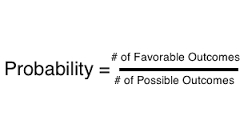• Save

# Basic Concepts of Probability with Exercise

Last year 9.3K ViewsA probability is a number that reflects the chance or likelihood that a particular event will occur. Probabilities can be expressed as proportions that range from 0 to 1, and they can also be expressed as percentages ranging from 0% to 100%. A probability of 0 indicates that there is no chance that a particular event will occur, whereas a probability of 1 indicates that an event is certain to occur. A probability of 0.45 (45%) indicates that there are 45 chances out of 100 of the event occurring.

Exercises:

1. (a) What is the probability of rolling a pair of dice and obtaining a total score of 9 or more? (b) What is the probability of rolling a pair of dice and obtaining a total score of 7?

2. A box contains four black pieces of cloth, two striped pieces, and six dotted pieces. A piece is selected randomly and then placed back in the box. A second piece is selected randomly. What is the probability that:
a. both pieces are dotted?
b. the first piece is black and the second piece is dotted?
c. one piece is black and one piece is striped?

3. A card is drawn at random from a deck. (a) What is the probability that it is an ace or a king? (b) What is the probability that it is either a red card or a black card?

4. The probability that you will win a game is 0.45. (a) If you play the game 80 times, what is the most likely number of wins? (b) What are the mean and standard deviation of a binomial distribution with ? = 0.45 and N = 80?

5. A fair coin is flipped 9 times. What is the probability of getting exactly 6 heads?

6. When Susan and Jessica play a card game, Susan wins 60% of the time. If they play 9 games, what is the probability that Jessica will have won more games than Susan?

7. You flip a coin three times. (a) What is the probability of getting heads on only one of your flips? (b) What is the probability of getting heads on at least one flip?

8. A test correctly identifies a disease in 95% of people who have it. It correctly identifies no disease in 94% of people who do not have it. In the population, 3% of the people have the disease. What is the probability that you have the disease if you tested positive?

9. A jar contains 10 blue marbles, 5 red marbles, 4 green marbles, and 1 yellow marble. Two marbles are chosen (without replacement). (a) What is the probability that one will be green and the other red? (b) What is the probability that one will be blue and the other yellow?

10. You roll a fair die five times, and you get a 6 each time. What is the probability that you get a 6 on the next roll?

Aswers:

1) (a) 5/18 = .278

2) (b) 1/6 = .167

3) (a) 2/13 = .154

4) (b) mean = 36; SD = 4.24

5) .164

7) (b) 7/8 = .875

9) (a) 2/19 = .105